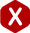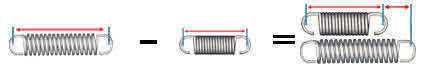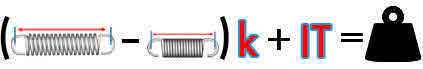<# Coil Tension Springs - Extended Length

#### Definition

##### The extended length of a coil tension spring is the total length of the extension spring once it is extended by a pulling load or force.

Coil tension springs have hooks on the ends because they are meant to be pulled on. This way one of the hooks is installed into a stationary object while the other is installed onto the object that will be applying the force or load. This object will extend the spring once a load is applied therefore making it reach a desired extended length. To calculate the extended length of your spring you must divide the load by the tension spring's rate and add the total to the spring’s length inside hooks. This is an easy task when you realize that the formula consists of the formula to calculate distance traveled and you simply add the length inside hooks to get the total extended length. This formula is provided below:

#### Extended Length Formula

L ÷ k + LIH = LL
• k = Rate
• LIH = Length Inside Hooks
• LL = Loaded / Extended Length

#### DiagramRemember that when designing your coil tension spring the point is for it to return to it’s pre-loaded position once the load is released. This means that you will have to know the amount of load you need in order to reach that extended length as well as the constant rate of force and initial tension.

To know how much distance of travel you will need your spring to extend in order to reach your desired coil spring extended length simply subtract the length inside hooks from the extended length as shown in the formula and diagram below.

#### Distance Traveled Formula

LL – LIH = DT
• LIH = Length Inside Hooks
• DT = Distance Traveled

#### DiagramTo calculate the load you need in order to reach your desired coil tension spring extended length you will subtract the length inside hooks from the extended length, multiply the product by your spring rate and add the amount of initial tension as the formula below shows.